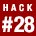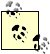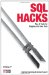# Hack 28. Avoid Dividing by ZeroNULLIF is a little-known function that returns NULL if its arguments are equal. It is handy for sidestepping a divide-by-zero exception.

The expression NULLIF(x,y) will give you x when x and y are different. If x and y are the same you get NULL.

Suppose you need to get a ratio from a pair of numbers but the divisor may be zero, as shown in Table 5-6.

Table 5-6. The stats table

page impressions clicks
index.htm 1,000 10
page1.htm 0 0
page2.htm 500 10

To calculate the click-through ratio (CTR) for each page you divide the number of clicks by the number of page impressions and multiply by 100. Because page1.htm was offline, it received zero page impressions, so the CTR calculation will give a divide-by-zero error. This example is from SQL Server (other databases give a similar error):

```1> SELECT page, 100.0*clicks/impressions FROM stats;
2> GO
page
-------------------- ----------------------------
index.htm 1.000000000000
Msg 8134, Level 16, State 1, Server TINYVAIO, Line 1
Divide by zero error encountered.```MySQL fails silently, returning NULL rather than a divide-by-zero error.

You can get around this problem with NULLIF to produce a NULL value when the number of impressions is zero:

```1> SELECT page, 100.0*clicks/NULLIF(impressions,0) FROM stats;
2> GO
page
-------------------- ----------------------------
index.htm 1.000000000000
page1.htm NULL
page2.htm 2.000000000000```

In SQL, any number divided by NULL gives NULL and no error is generated.

## IFNULL and ISNULL and NULLIF

IFNULL is a MySQL function. It is similar to COALESCE and NVL. IFNULL returns the first argument unless it is NULL, in which case it returns the second argument.

ISNULL is another MySQL function. ISNULL returns 1 when the input is NULL, and NULL otherwise.

NULLIF, as demonstrated in this hack, is an ANSI standard SQL function supported by all of the major SQL vendors.SQL Hacks
ISBN: 0596527993
EAN: 2147483647
Year: 2004
Pages: 147